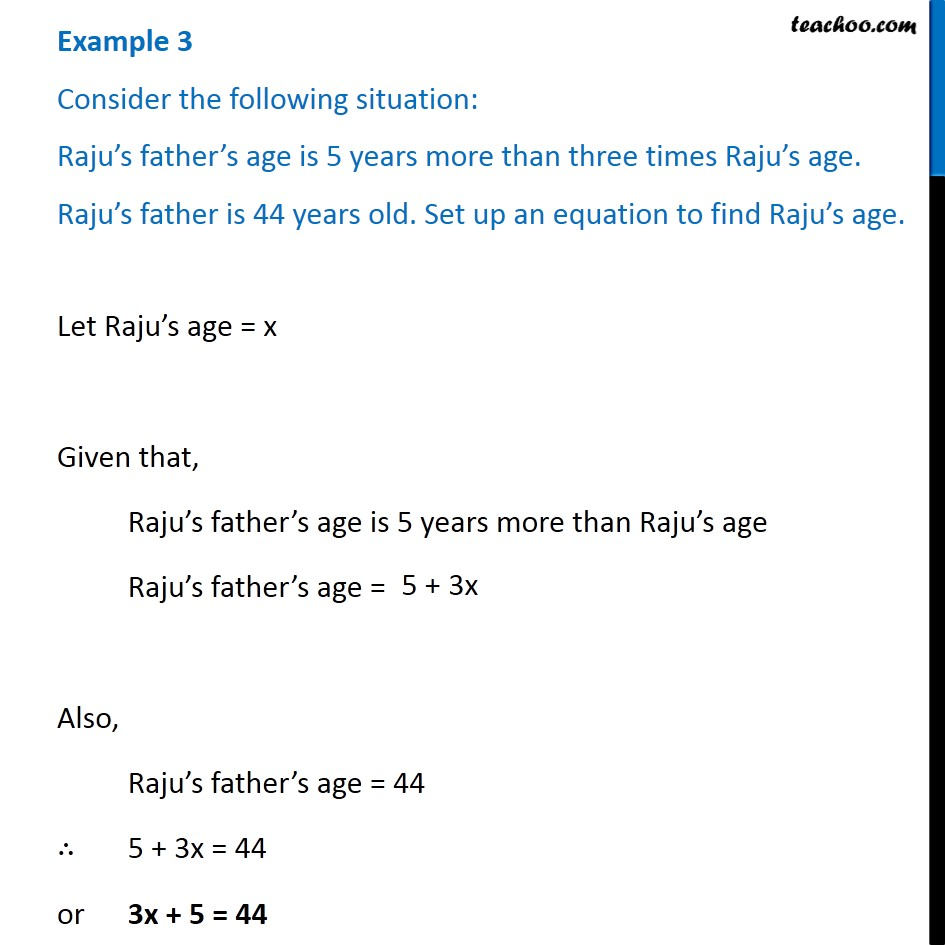Making equations from statements

Chapter 4 Class 7 Simple Equations
Concept wise### Transcript

Example 3 Consider the following situation: Raju s father s age is 5 years more than three times Raju s age. Raju s father is 44 years old. Set up an equation to find Raju s age. Let Raju s age = x Given that, Raju s father s age is 5 years more than Raju s age Raju s father s age = Also, Raju s father s age = 44 5 + 3x = 44 or 3x + 5 = 44